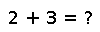# median

median(v[, region])

Compute the median of whole array `v`, or optionally along the dimensions in `region`. For even number of elements no exact median element exists, so the result is equivalent to calculating mean of two median elements. `NaN` is returned if the data contains any `NaN` values. For applications requiring the handling of missing data, the `DataArrays` package is recommended.

## Examples

``````julia> xsum = rand(5)
median(xsum)
0.6850542627340954``````
``````julia> A = rand(3)
3-element Array{Float64,1}:
0.722853
0.606612
0.846898
julia> median(A)
0.6557513931743997``````

Checking you are not a robot: